## Simpson and trapezoidal rule for definite integral...

Hi experts, I know built in command to solve definite integral by using simpson and trapezoidal rule but i need to show step which is given in the example to satisfy his students of grade 12. Please help me to solve this example## Why does my Maple animation only work for 120 fram...

I have an animation in Maple that only works if I use the option frames=120, or anything >120. Anything less and it gives the error "Error, (in plots/animate) points cannot be converted to floating-point values". I don't understand why this restriction is happening, any advice is appreciated.

## Is there a better way to impletment a transparent...

transparent_background_in_plots.mw

Is there a better way to impletment a transparent background when genereating plots?

Here I generate a plot with a yellow background.

plot(sin(x), x = 0 .. 4*Pi, background = yellow);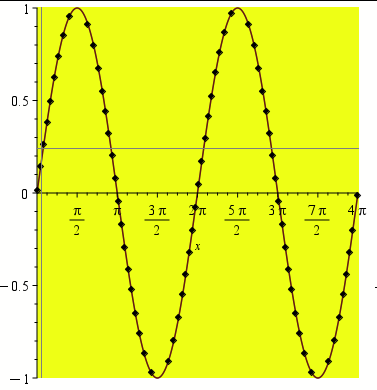I want the background to be completely transparent instead of yellow.

plot(sin(x), x = 0 .. 4*Pi, background = none);

Error, (in plot) Unable to find image file, none

none is not an accepted color, so an error is returned. Is there such a color as "clear" that is fully transparent? Is there a better way to impletment a transparent background when genereating plots?

## The NormalSeries of maple is different with textb...

As we know, when every Gi is normal in G, then the series is called a normal series:

`cs := CompositionSeries(DihedralGroup(8))`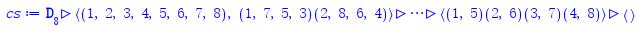We can draw it:

`DrawSubgroupLattice(DihedralGroup(8), labels = ids, highlight = cs)`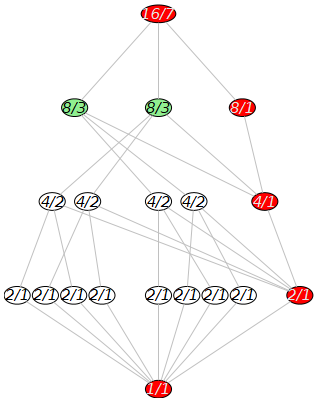And all Gi is normal in G:

`IsNormal~(cs, DihedralGroup(8))`

[true, true, true, true, true]

But why `type(cs, 'NormalSeries')` will get `false`.. It is a bug or do I have a misunderstanding?

## How to call parent class constructor from sub clas...

I am still struggling understanding Maple Object  inheritance due to lack of good examples and sparse documenation.

This is what I am trying to do. I have base class Person, and then make Employee class which extends Person class and adds new field.

The constructor to the Employee class then needs to call the constructor to the Person class (its base class) in order to initialize it. The base class constuctor takes care of initialization the fields in the base class.

I do not know how to do this. Actually it is not clear to me how the proto argument gets into play in all of this. I could not find one example that explains how to use proto with inheritance.

I am trying to implement this python example. I will show the code for the Python, and my Maple translation. The part I do not know how to do, it how to call the constructor for the base class from the sub class.  If I do not do this, then the fields of the base class will remain unitilized.

Python example is from https://www.geeksforgeeks.org/python-access-parent-class-attribute/

```# parent class
class Person( object ):

# __init__ is known as the constructor
def __init__(self, name, idnumber):
self.name = name
self.idnumber = idnumber

def display(self):
print(self.name)
print(self.idnumber)

# child class
class Employee( Person ):
def __init__(self, name, idnumber, salary):
self.salary = salary

# invoking the constructor of the parent class
#----->>>>> HOW TO DO THIS IN MAPLE?
Person.__init__(self, name, idnumber)

def show(self):
print(self.salary)

# creation of an object
# variable or an instance
a = Employee('Rahul', 886012, 30000000)

# calling a function of the
# class Person using Employee's
# class instance
a.display()
a.show() ```

Here is the Maple code

```restart;
person:=module()
option object;
#I made these exported instead of local for ease of printing from outside while
#debugging , that is all.. These should otherwise be local

export idnumber::integer:=0;
export name::string:="";

export ModuleCopy::static:= proc( _self, proto, name::string, idnumber::integer, \$)
_self:-idnumber := idnumber;
_self:-name := name;
end proc;

end module;
#---- extend the above class

employee:=module()
option object(person);
export salary::integer:=0;

ModuleCopy::static:= proc( _self, proto, name::string, idnumber::integer, salary::integer , \$)
_self:-salary := salary;
# invoking the constructor of the parent class
# How to do that in Maple?

#I could always manually do this. But I do not want to duplicate the code
#done by the base class constructor.
#_self:-name := name;
#_self:-idnumber := idnumber;

end proc;

end module;

```

How does one do the Python example in Maple?

Someone should write a book on programming using OOP in Maple. I'll buy a copy in advance.

Maple 2022.1

## Contour Plot from list...

Please, how do plot the contour plot of im(qq) vs Re(qq) from the list below:

qq= [2.106333379+.6286420119*I, 2.106333379-.6286420119*I, 4.654463885, 7.843624703, 10.99193295, 14.13546782, 17.27782732, 20.41978346, 23.56157073, 26.70327712, 29.84494078, 32.98658013, 36.12820481, 39.26982019, 42.41142944, 45.55303453, 48.69463669, 51.83623675, 54.97783528, 58.11943264, 61.26102914]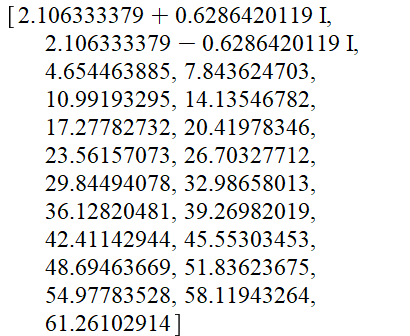## Minimum. ...

How does one compute the location of the minimum algebraically (rather than numerically) of the following expression:
F:=((z^2+(z^2+2*z+2)^(1/2)*(z^2+1)^(1/2)+z+1)^(1/2)*(-z^2+(z^2-2*z+2)^(1/2)*(z^2+1)^(1/2)+z-1)^(1/2)-(z^2+(z^2-2*z+2)^(1/2)*(z^2+1)^(1/2)-z+1)^(1/2)*(-z^2+(z^2+2*z+2)^(1/2)*(z^2+1)^(1/2)-z-1)^(1/2)+2*z)/(2*z^2+2);

## I need the solve in Maple...

Write a Maple procedures called “biggest4” and “smallest4” to find
a) the biggest
b) the smallest
of the given numbers  a = -1/3, b = 0, c = 7/3, d = -2

i guess i need to use end proc for programing but i dont understand:

## Print a set of graphs via for loop...

I have set of graphs say with names

Graph1,Graph2,..., Graph50.

1) I want to print all these using a single for.

2) I want to check the Isomorphic property of which two are all Isomorphic pairwise using a for loop say

Or if any other methods kind help with suitable advice.

## How do I solve a initial value problem in Maple...

i need to use two different methods to solve the initial value problem. I tried but didnt find the answer

y" + 4y' + 4y = sin(x), y(0) = 1 , y'(0) = 0

y" + 4*diff(y(x), x) + 4*y(x) = sin(x)

dsys1 := {diff(y(x), x, x) + diff(y(x), x) + 4*y(x) = sin(x), y(0) = 1, D(y)(0) = 0};
dsol1 := dsolve(dsys1, numeric, method = gear);
dsol1(0);

## How do I solve a equation in Maple?...

y = ax^3 + bx^2 + cx + d this is curve and is passing through the points (−1,−4),(1,0),(2,0),(3,−4).
1) Find the values of 𝑎,𝑏,𝑐 and 𝑑.
2) Plot the curve and the points given on the same axis.

I tried solving with derivative of the curve but i didnt find the exact values.

## how to give variable a type and also make it stat...

I need to make my base class local variable static, so that when extending the class, the subclass will share these variable and use their current values as set by the base class. If I do not make them static, then the base class when extended, will get fresh instance of these variable, losing their original values, which is not what I want.

To do this, one must make the base class variables static

This works, but now I do not know the syntax where to put the type on the variable.

I can't write   local m::integer::static; nor local m::static::integer;

I could only write local m::static; but this means I lost the ability to have a type on the variable and lost some of the type checking which is nice to have in Maple. From Maple help: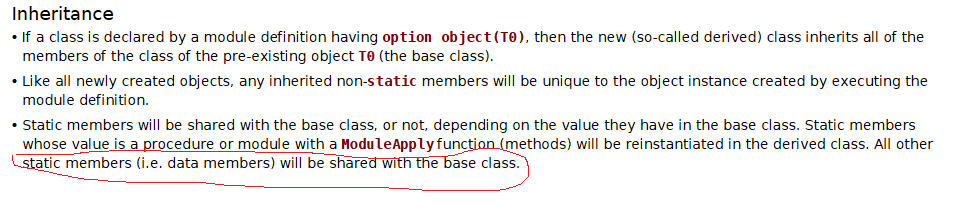Here is example

```restart;

base_class:=module()
option object;
local n::static;  #I want this type to ::integer also. But do not know how

export set_n::static:=proc(_self,n::integer,\$)
_self:-n := n;
end proc;

export process::static:=proc(_self,\$)
local o;
o:=Object(sub_class);
o:-process();
end proc;
end module;

sub_class:=module()
option object(base_class);
process:=proc(_self,\$)
print("in sub class. _self:-n = ",_self:-n);
end proc;
end module;

o:=Object(base_class);
o:-set_n(10);
o:-process()

"in sub class. _self:-n = ", 10```

The above is all working OK. I just would like to make n in the base class of type ::integer as well as ::static

Is there a syntax for doing this?

## Newton Raphson method for grade 12...

Could any one please guide me how to show this solution by using Newton Raphson method. I need to show this solution step by step as shown in example.## Need to view solution step by step...

Hi Community, I have solved the some basic expression by using built in command to get solution. Now i am interested to view the solution stp by step to show  my students of grade 10.

 >>(1)
 >(2)
 >(3)
 >(4)
 >(5)
 >(6)
 >(7)
 >(8)
 >(9)
 >(10)
 >(11)
 >Solving a system of equations:

 >(12)
 >(13)
 >(14)
 >(15)
 >>(16)
 >